# Control Statements in JavaChallenge Inside! : Find out where you stand! Try quiz, solve problems & win rewards!

## Overview

Control Statements are the base of any programming language. Using control statements we implement real world scenarios in programs. There are three types of Control Statements in Java:

• Decision-Making Statements
• Loop Statements
• Jump or Branch Statements

Decision making statements execute a piece of code based on some condition. Looping Statements execute a piece of code repeatedly until a condition becomes false. Jump or branch statements help in transferring the control of the flow of execution to a specific point in the code.

## Scope

• Discuss various types of Control Statements in Java
• Explain Decision Making Statements such if, if-else, if-else-if ladder and switch statements
• Illustrate Loop Statements such for, while, do-while and foreach statements.
• Explain Jump or Branching Statements such as break, while and return statements.

## Introduction to Control Statements in Java

In Java, the compiler parses the application code sequentially from top to bottom. The code statements are executed according to the order in which they appear.

It's a very difficult task to model real-world problems in the code if we have no control statements. Control statements in Java are one of the fundamental features of Java which provides a smooth flow of the program.

These statements decide whether a specific part of the code will be executed or not. So it is used to control the flow of the program by executing the code on a conditional basis.

Before moving ahead with the article, you should read the following Java Programming topics:

## Types of Control Flow Statements

There are different types of control statements in Java for different conditions. We can divide control statements in Java into three major types:

1. Decision-making statements
2. Loop statements
3. Jump/Branching statements

In this section, we’ll go into detail with examples of each of these statements with their uses and significance.

## Decision-Making Statements

Decision-making statements in Java are similar to making a decision in real life, where we have a situation and based on certain conditions we decide what to do next. For example, if it's raining outside then we need to carry an umbrella. In programming also, we have some situations when we need a specific code to be executed only when a condition is satisfied. The decision control statements help us in this task by evaluating a boolean expression and accordingly controlling the flow of the program.

There are four types of decision-making statements in Java:

### 1. if Statement

These are the simplest and yet most widely used control statements in Java. The if statement is used to decide whether a particular block of code will be executed or not based on a certain condition. If the condition is true, then the code is executed otherwise not.

Let's see the execution flow of the if statement in a flow diagram: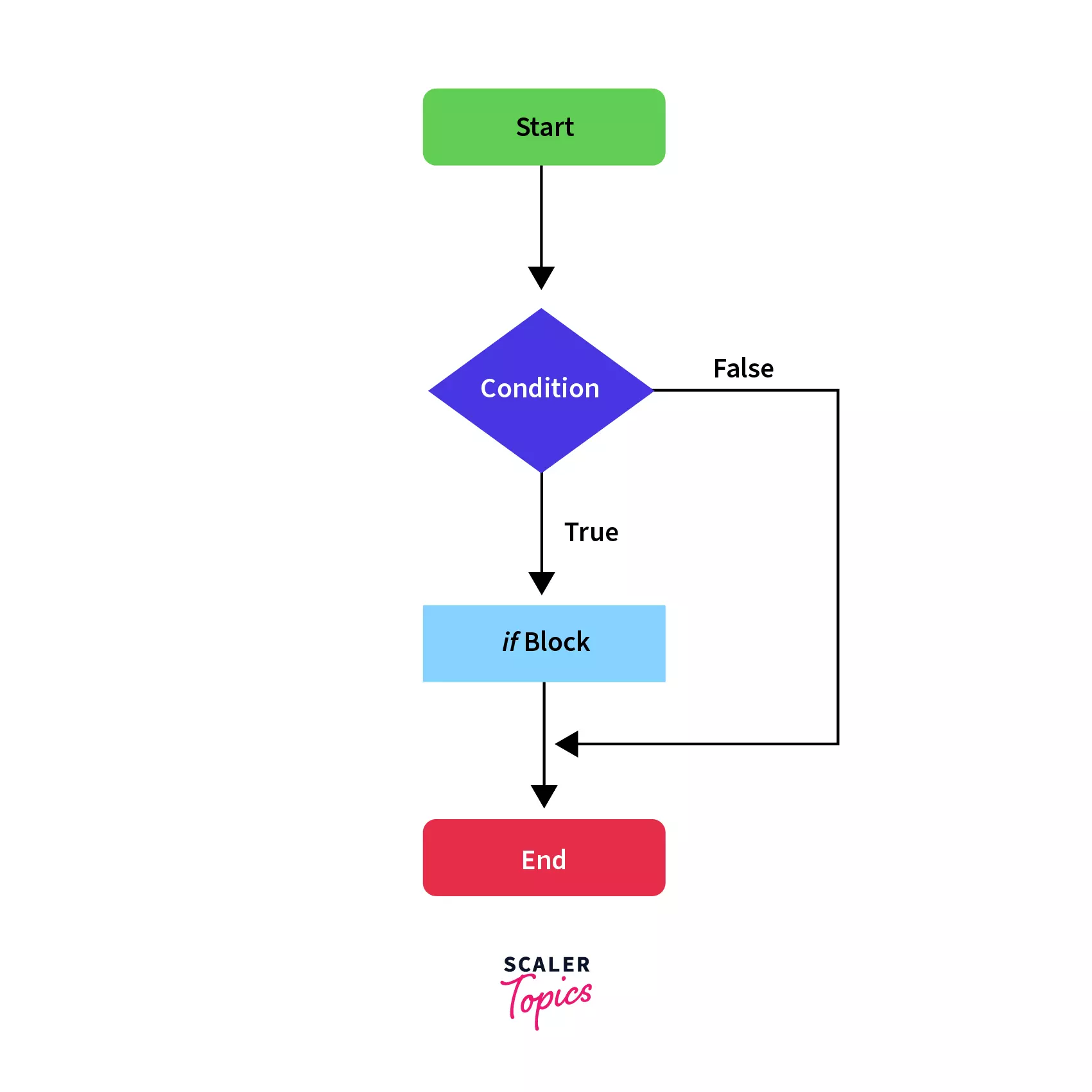In the above flow diagram, we can see that whenever the condition is true, we execute the if block otherwise we skip it and continue the execution with the code following the if block.

The syntax and execution flow of the if statement is as follows:

Syntax:

``````if(condition) {
// block of code to be executed if the condition is true
}
``````

The if statement evaluates the given condition and if the condition is true, then the code inside the if block (marked by curly brackets) is executed. If we do not give curly brackets after the if statement then only the immediate statement following the if statement is considered to be inside the if block.

For example:

``````if(condition)
statement1;
statement2;
``````

Here statement1 will be executed only if the condition is true. The statement2 is executed irrespective of the condition being true or false.

Example:

Let’s say that we want to dynamically load a screen on the user browser based on its role in our system. If the role is admin, then only we'll load the screen otherwise not. Let’s try to code this now:

``````String role = "admin";
}
``````

Output:

``````Admin screen is loaded
``````

Here, since the value of the role variable is admin, the print statement inside if is executed. If we change the value of the role to any other value, then the condition will be false and we get no output.

### 2. if-else Statement

The if statement is used to execute a block of code based on a condition. But if the condition is false and we want to do some other task when the condition is false, how should we do it?

That's where else statement is used. In this, if the condition is true then the code inside the if block is executed otherwise the else block is executed.

Let's see the execution flow of the if-else statement in a flow diagram: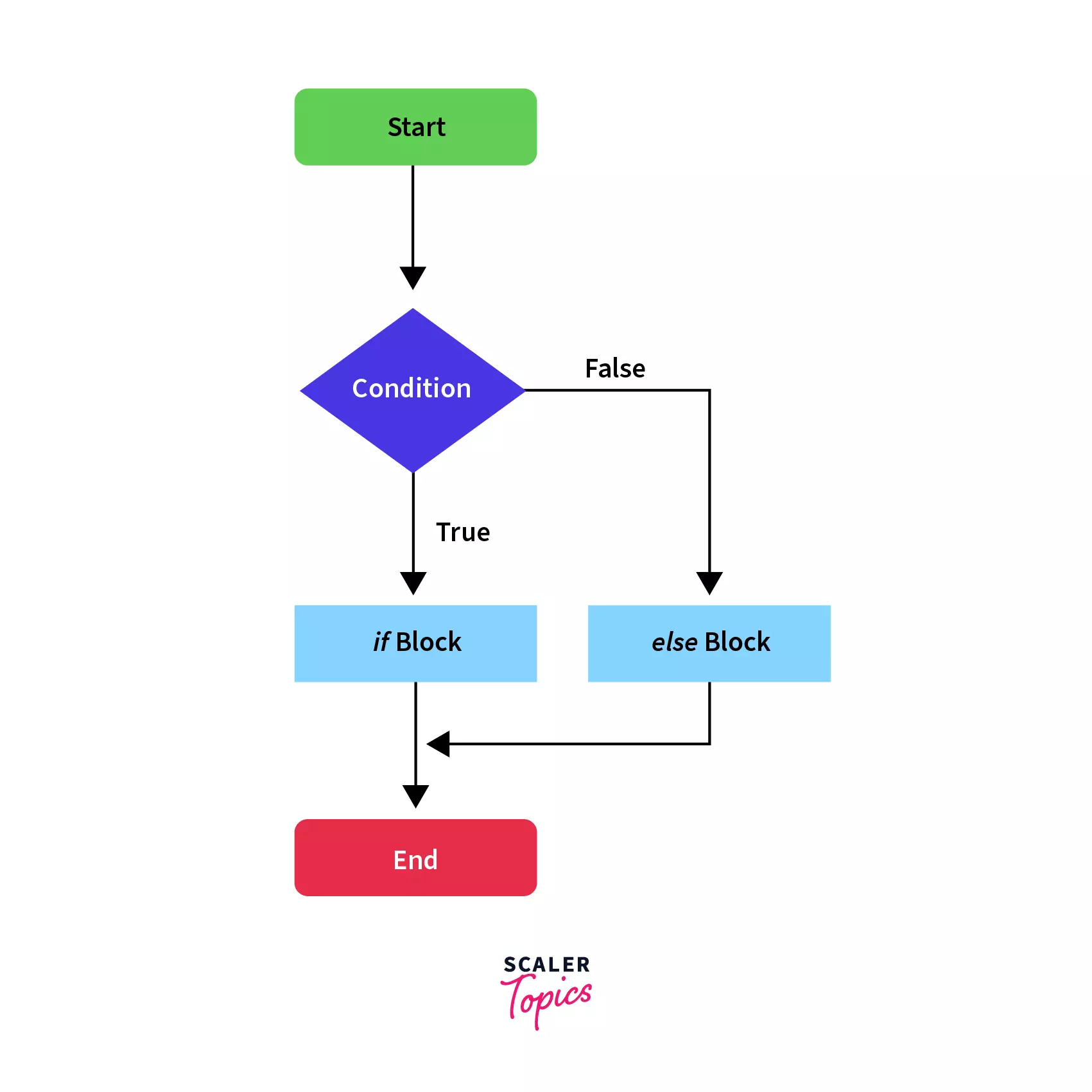The above flow diagram is similar to the if statement, with a difference that whenever the condition is false, we execute the else block and then continue the normal flow of execution.

The syntax and execution flow of the if-else statement is as follows:

Syntax:

``````if (condition) {
// If block executed when the condition is true
}
else {
// Else block executed when the condition is false
}
``````

Now, if the condition is false, the else block is executed and the if block code is skipped. This is one example of controlling the flow of a program through control statements in java.

Example:

Continuing our previous example, let’s say that if the role is not admin, then we'll load the user screen. Let’s try to implement this through if-else:

``````String role = "user";
}
else {
}
``````

Output:

``````User screen is loaded
``````

Here, since the value of role is not admin, the condition is false and hence the else block is executed and we get User screen is loaded as output.

### 3. Nested if-else Statement

Java allows us to nest control statements within control statements. Nested control statements mean an if-else statement inside other if or else blocks. It is similar to an if-else statement but they are defined inside another if-else statement.

Let us see the syntax of a specific type of nested control statements where an if-else statement is nested inside another if block.

Syntax:

``````if (condition) {
// If block code to be executed if condition is true
if (nested condition) {
// If block code to be executed if nested condition is true
}
else {
// Else block code to be executed if nested condition is false
}
}
else {
// Else block code to be executed if condition is false
}
``````

Explanation:

Here, we have specified another if-else block inside the first if block. In the syntax, you can see the series of the blocks executed according to the evaluation of condition and nested condition. Using this, we can nest the control flow statements in Java to evaluate multiple related conditions.

Let's see the execution flow of the above-mentioned nested-if-else statement in a flow diagram: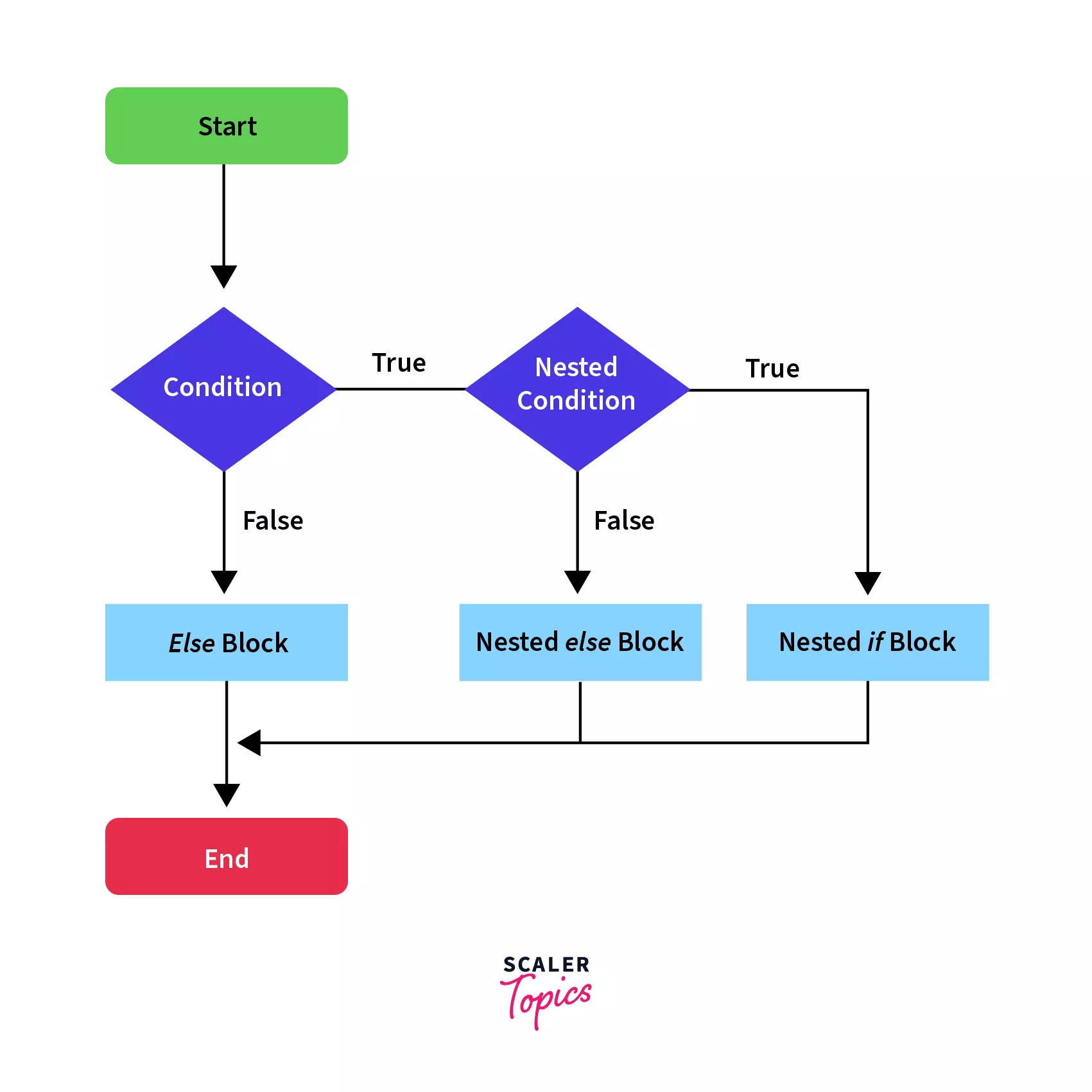Example:

Let’s say that we want to know which floor of the mall a person needs to go to do shopping based on the age and gender of the person. Let’s see the implementation of this example through nested-if:

``````int age = 20;
String gender = "male";
if (age > 18) {
if (gender == "male") {
// person is a male
System.out.println(
"You can shop in the men's section on the 3rd Floor"
);
} else {
// person is a female
System.out.println("You can shop in the women's section on 2nd Floor");
}
} else {
// person is not an adult
System.out.println("You can shop in the kid's section on 1st Floor");
}
``````

Output:

``````You can shop in the men's section on the 3rd Floor
``````

In this, the if statement is followed by multiple else-if blocks. We can create a decision tree by using these control statements in Java in which the block where the condition is true is executed and the rest of the ladder is ignored and not executed. If none of the conditions is true, the last else block is executed, if present.

Let's see the execution flow of the if-else ladder in a flow diagram: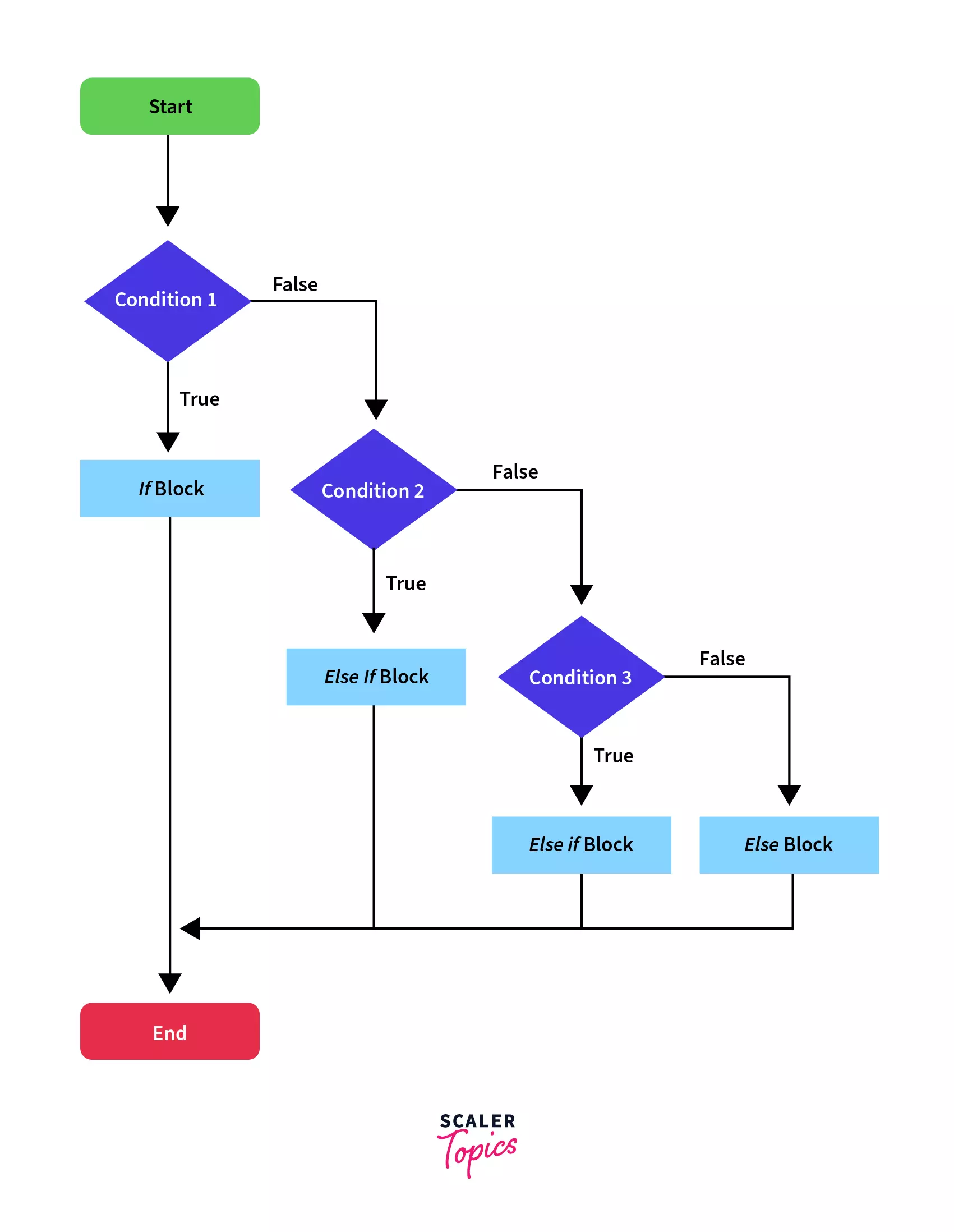As you can see in the above flow diagram of the if-else ladder, we execute the if block if the condition is true, otherwise if the condition is false, instead of executing the else block, we check other multiple conditions to determine which block of code to execute. If none of the conditions are true, the last else block, if present, is executed.

The syntax and execution flow of the if-else-if ladder statement is as follows:

Syntax:

``````if(condition1) {
// Executed only when the condition1 is true
}
else if(condition2) {
// Executed only when the condition2 is true
}
.
.
.
.
else {
// Executed when all the conditions mentioned above are true
}
``````

Example:

Let’s say that we have a browser-specific code, where we want to execute a code depending upon the browser the user is using. Let’s try to implement this in the code:

``````String browser = "chrome";
if(browser == "safari") {
System.out.println("The browser is safari");
}
else if(browser == "edge") {
System.out.println("The browser is edge");
}
else if(browser == "chrome"){
System.out.println("The browser is chrome");
}
else {
System.out.println("Not a supported browser");
}
``````

Output:

``````The browser is chrome
``````

Explanation:

As you can see, the first two conditions are not true. Hence, the first two blocks are not executed. The third condition is true and hence the third block of code is executed giving the output: The browser is chrome. Following this block, all other blocks (the last else block) are ignored.

Here we can write the browser-specific implementation in the equivalent block, and if none of the supported browsers are encountered then we can also give an error in the last else block.

### 5. switch Statement

Switch statements are almost similar to the if-else-if ladder control statements in Java. It is a multi-branch statement. It is a bit easier than the if-else-if ladder and also more user-friendly and readable. The switch statements have an expression and based on the output of the expression, one or more blocks of codes are executed. These blocks are called cases. We may also provide a default block of code that can be executed when none of the cases are matched similar to the else block.

The syntax and execution flow of the switch statement is as follows:

Syntax:

``````switch (expression) {
case value1:
//code block of case with value1
break;
case value2:
//code block of case with value2
break;
.
.
case valueN:
//code block of case with valueN
break;
default:
//code block of default value
}
``````

There are certain points that one needs to be remembered while using switch statements:

• The expression can be of type String, short, byte, int, char, or an enumeration.
• We cannot have any duplicate case values.
• The default statement is optional.
• Usually, the break statement is used inside the switch to terminate a statement sequence.
• The break statement is optional. If we do not provide a break statement, the following blocks will be executed irrespective of the case value. This is known as the trailing case.

Let's see the execution flow of the switch statement in a flow diagram: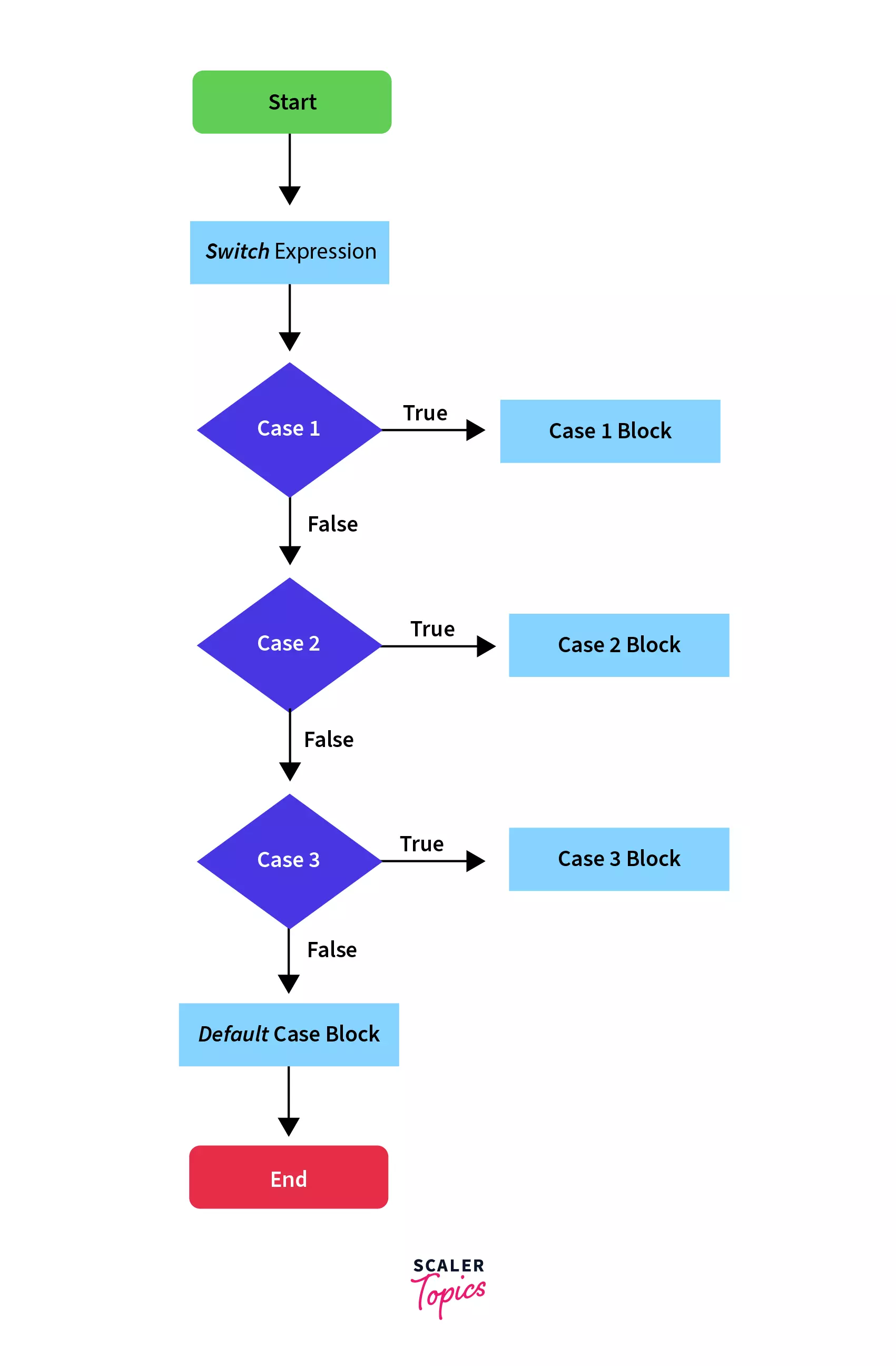In the above flow diagram, we have a switch expression and we match the output of the expression through a series of case blocks. Whichever case matches the output, its block is executed and execution skips to the end of the switch; otherwise, if none of the cases matches, the default block is executed.

Here, we have multiple case statements and each case code block is followed by a break statement to stop the execution to that case only.

Example:

If we take the previous example, we can easily implement that using switch statements:

``````String browser = "chrome";
switch (browser)
{
case "safari":
System.out.println("The browser is Safari");
break;
case "edge":
System.out.println("The browser is Edge");
break;
case "chrome":
System.out.println("The browser is Chrome");
break;
default:
System.out.println("The browser is not supported");
}
``````

Output:

``````The browser is chrome
``````

In this example, the case with the value “chrome” is matched and hence its block is executed. If we do not give a break statement in this block, the trailing blocks(default block in this example) will also be executed. Also, it's worthwhile to notice that the switch statement made the code more readable and cleaner.

Let’s see the output if we omit the break statement:

Code:

``````String browser = "chrome";
switch (browser)
{
case "safari":
System.out.println("The browser is Safari");
case "edge":
System.out.println("The browser is Edge");
case "chrome":
System.out.println("The browser is Chrome");
default:
System.out.println("The browser is not supported");
}
``````

Output:

``````The browser is Chrome
The browser is not supported
``````

We can see that the default block is also executed. It is due to the following reason: Once a case value is matched, all the following blocks of code are executed until a break statement or the end of the switch statement is encountered.

This is not the expected behavior and hence we should use the break statement. We’ll learn more about the break statement in the next sections.

## Loop Statements

Java provides a set of looping statements that executes a block of code repeatedly while some condition evaluates to true. Looping control statements in Java are used to traverse a collection of elements, like arrays.

Java provides the following looping statements:

### 1. while Loop

The while loop statement is the simplest kind of loop statement. It is used to iterate over a single statement or a block of statements until the specified boolean condition is false.

The while loop statement is also called the entry-control looping statement because the condition is checked prior to the execution of the statement and as soon as the boolean condition becomes false, the loop automatically stops.

You can use a while loop statement if the number of iterations is not fixed. Normally the while loop statement contains an update section where the variables, which are involved in while loop condition, are updated.

Let's see the execution flow of the while loop statement in a flow diagram: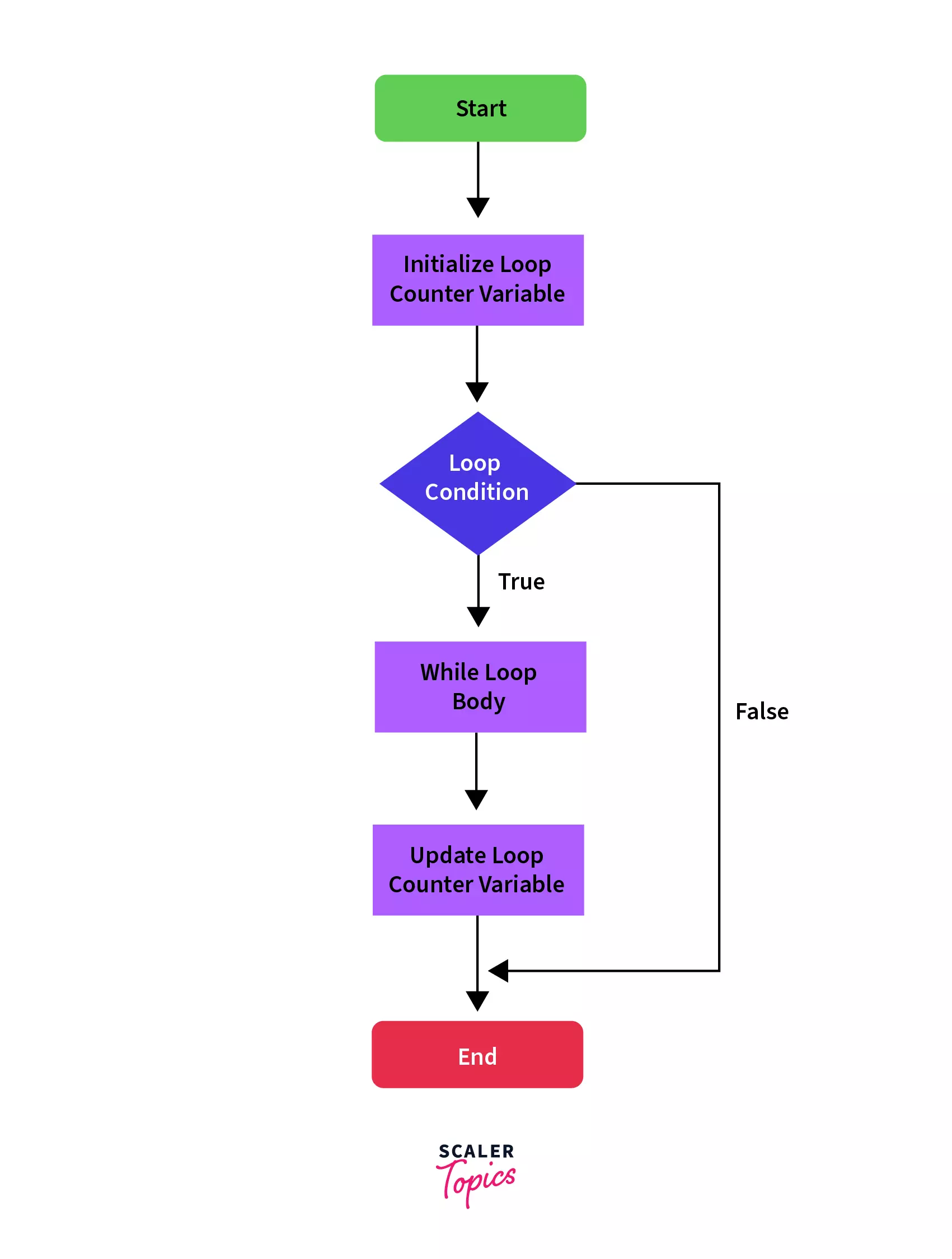In the above flow diagram of a while loop:

• We initialize a loop counter variable. After that, we check the loop condition and if it's true, then the body of the loop is executed followed by the update of the counter variable.
• The control then again switches back to the loop condition and the cycle continues till the condition is false and we execute the statements outside the loop body.

The syntax and execution flow of the while loop statement is as follows:

Syntax:

``````while (condition)
{
// code block to be executed
}
``````

Example:

Let's say we want to print the numbers from 10 to 1 in decreasing order. Let's implement this through a while loop.

``````public class WhileLoopDemo {

public static void main(String args[]) {
int num = 10;
while (num > 0) {
System.out.println(num);

// Update Section
num--;
}
}
}
``````

Output:

``````10
9
8
7
6
5
4
3
2
1
``````

Here, we have used the loop condition as num > 0 and then at each iteration of the loop, we have decreased the value of the num variable by 1.

So the loop runs till the value of num becomes 1 from 10, and we get the desired output.

### 2. do-while Loop

The Java do-while loop statement works the same as the while loop statement with the only difference being that its boolean condition is evaluated post first execution of the body of the loop. Thus it is also called exit controlled looping statement. You can use a do-while loop if the number of iterations is not fixed and the body of the loop has to be executed at least once.

Let's see the execution flow of the do-while loop statement in a flow diagram: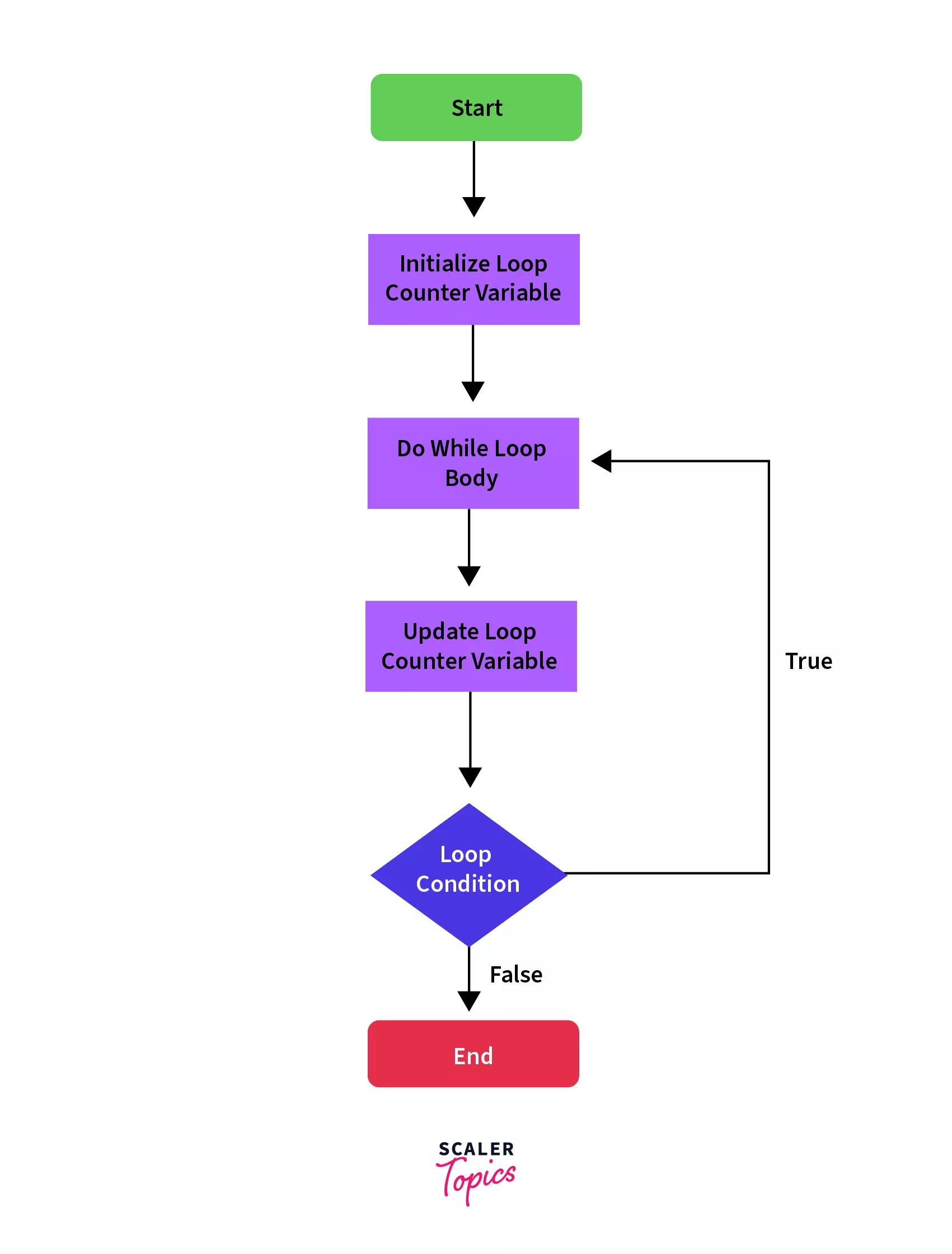In the above flow diagram of a do-while loop:

• We also initialize a counter variable, but instead of checking the loop condition at the start, the body of the loop is executed.
• After the completion of the loop body, we check the loop condition and continue to execute the loop body till the condition is false when we come out of the loop and execute the rest of the code.

The syntax and execution flow of the do-while loop statement is as follows:

Syntax:

``````do
{
// code block to be executed
} while (condition);
``````

Example:

Let's try to use the same example of printing the number in decreasing order through a do-while loop:

``````public class Main {

public static void main(String args[]) {
int num = 10;
do {
System.out.println(num);
num--;
} while (num > 0);
}
}
``````

Output:

``````10
9
8
7
6
5
4
3
2
1
``````

The implementation and output are almost similar, with the change that, even if the value of the num initially is less than 1. We'll get at least one print statement with the value of the num as the output.

### 3. for Loop

Unlike the while loop control statements in Java, a for loop statement consists of the initialization of a variable, a condition, and an increment/decrement value, all in one line. It executes the body of the loop until the condition is false.

The for loop statement is shorter and provides an easy way to debug structure in Java. You can use the for loop statement if the number of iterations is known.

In a for loop statement, execution begins with the initialization of the looping variable, then it executes the condition, and then it increments or decrements the looping variable. If the condition results in true then the loop body is executed otherwise the for loop statement is terminated.

Let's see the execution flow of the for loop statement in a flow diagram: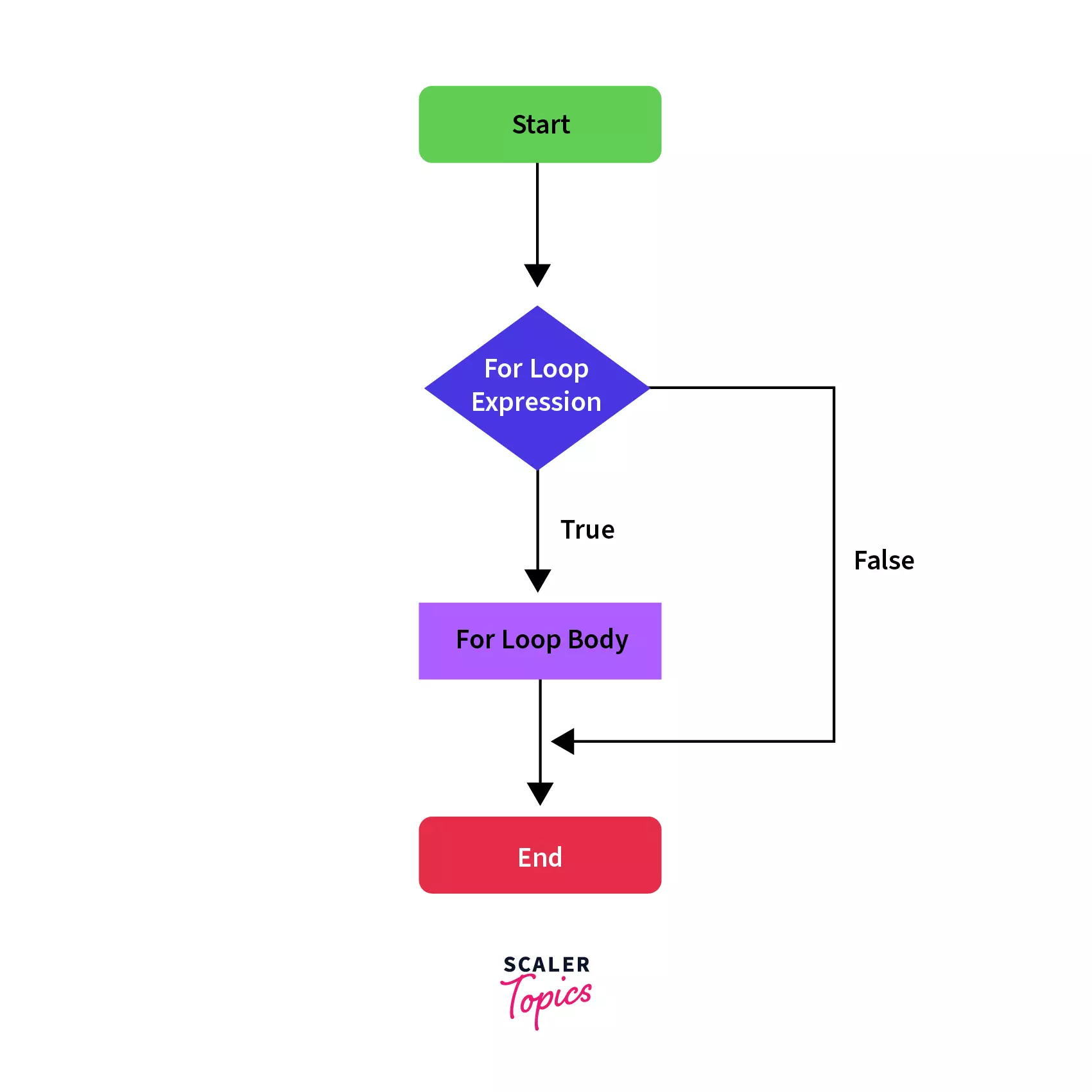As you can see in the above flow diagram, we have a for loop statement. In this statement, the loop condition is checked, and if the condition is true, the for loop body is executed until the condition is false and we continue with the normal flow of execution.

The syntax and execution flow of for loop statement is as follows:

Syntax:

``````for (initialization; condition; increment/decrement)
{
// code block to be executed if condition is true
}
``````

Example:

``````public class ForLoopDemo {

public static void main(String args[]) {
for (int num = 10; num > 0; num--) System.out.println(
"The value of the number is: " + num
);
}
}
``````

Output:

``````The value of the number is: 10
The value of the number is: 9
The value of the number is: 8
The value of the number is: 7
The value of the number is: 6
The value of the number is: 5
The value of the number is: 4
The value of the number is: 3
The value of the number is: 2
The value of the number is: 1
``````

### 4. for-each Loop

The for-each loop statement provides an approach to traverse through elements of an array or a collection in Java. It executes the body of the loop for each element of the given array or collection. It is also known as the Enhanced for loop statement because it is easier to use than the for loop statement as you don’t have to handle the increment operation. The major difference between the for and for-each loop is that for loop is a general-purpose loop that we can use for any use case, the for-each loop can only be used with collections or arrays.

In for-each loop statement, you cannot skip any element of the given array or collection. Also, you cannot traverse the elements in reverse order using the for-each loop control statement in Java.

Let's see the execution flow of the for-each loop statement in a flow diagram: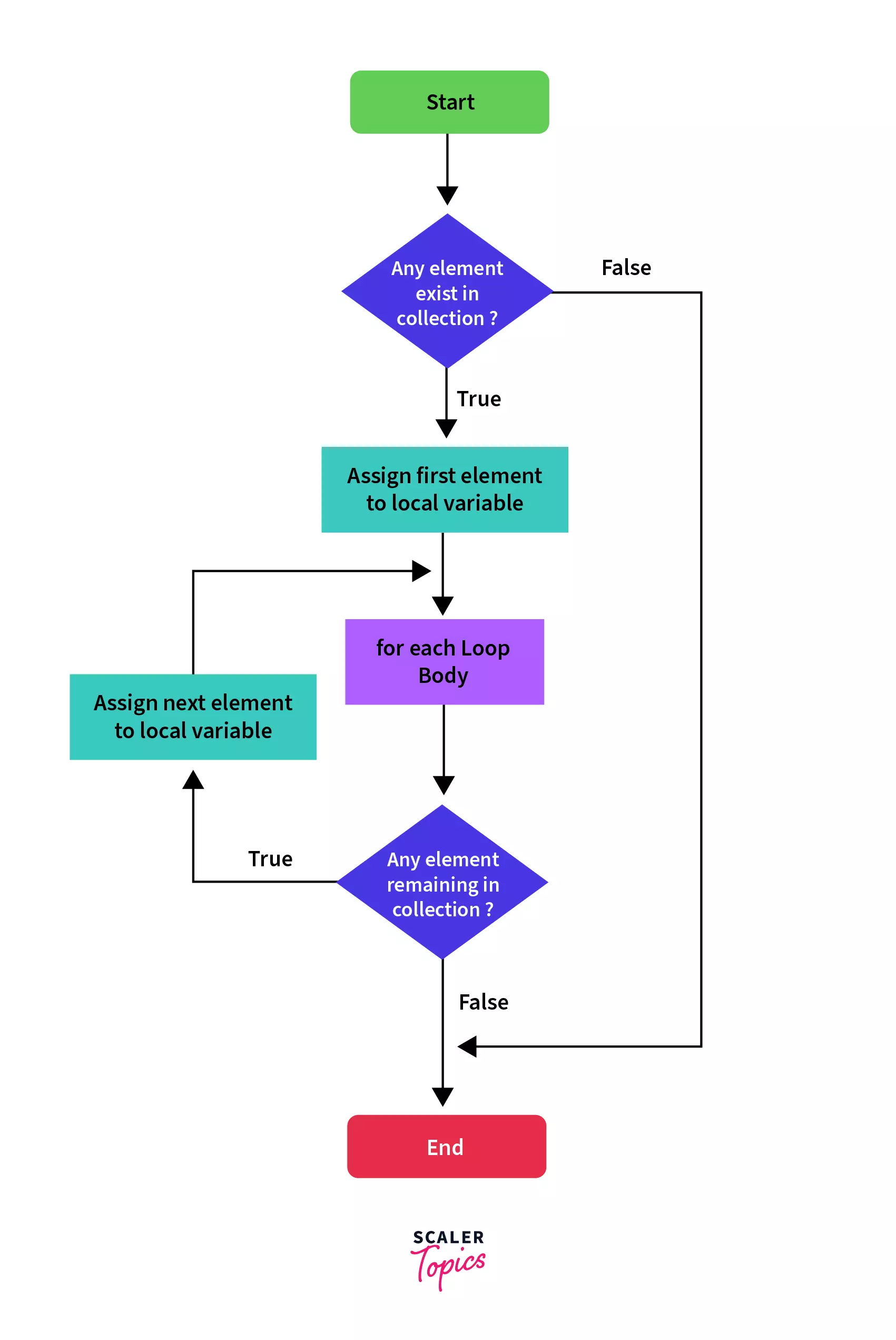In the above flow diagram of a for each loop:

• We check if the collection has any elements or not. If it has the elements, then the first element is assigned to the local variable mentioned in the for each expression, and the for each loop body is executed.
• After this, we again check if the collection has any remaining elements and this cycle continues till we have traversed all the elements.

The syntax and execution flow of for each loop statement is as follows:

Syntax:

``````for(dataType variableName : array | collection)
{
// code block to be executed
}
``````

Example:

``````public class ForEachLoopDemo {

public static void main(String args[]) {
int[] array = { 10, 9, 8, 7, 6, 5, 4, 3, 2, 1 };
System.out.println("Elements of the array are: ");
for (int elem : array) System.out.println(elem);
}
}
``````

Output:

``````Elements of the array are:
10
9
8
7
6
5
4
3
2
1
``````

## Jump/Branching Statements

Jump/Branching control statements in Java transfer the control of the program to other blocks or parts of the program and hence are known as the branch or jump statements.

Java provides us the following jump or branching statements:

### 1. Break Statement

The break statement as we can deduce from the name is used to break the current flow of the program. The break statement is commonly used in the following three situations:

• Terminate a case block in a switch statement as we saw in the example of the switch statement in the above section.
• To exit the loop explicitly, even if the loop condition is true.
• Use as the alternative for the goto statement along with java labels, since java doesn’t have goto statements.

The break statement cannot be used as a standalone statement in Java. It must be either inside a switch or a loop. If we try to use it outside a loop or a switch, JVM will give an error.

Let's see the execution flow of the break statement in a flow diagram: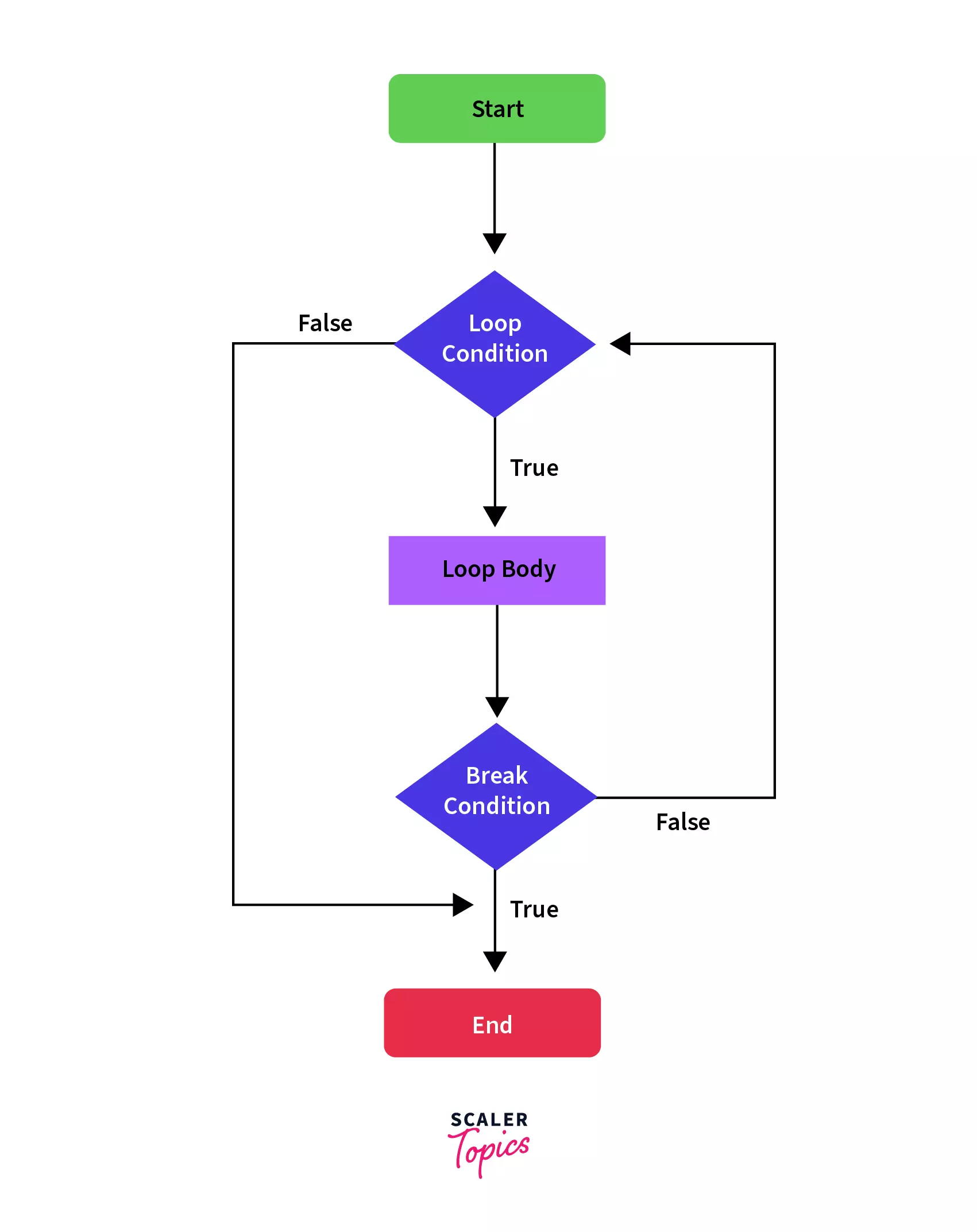In the above flow diagram of a break statement, whenever the loop body encounters a break statement, it stops the current flow of execution and jumps to the first statement out of the loop body.

The syntax of the break statement is as follows:

Syntax:

We have already seen how we use the break inside a switch. Let’s see the syntax in the case of a loop:

``````for(condition) {
// body of the loop
break;
}
while(condition) {
// body of the loop
break;
}
``````

Example 1:

``````for(int index = 0; index < 10; index++) {
System.out.println("The value of the index is: " + index);
if(index == 3) {
break;
}
}
``````

Output:

``````The value of the index is: 0
The value of the index is: 1
The value of the index is: 2
The value of the index is: 3
``````

As we can see the loop was terminated even when the condition of the loop was still true. This is how we can use the break statement in a loop.

Example 2:

In case, we have nested loops, the break statement will only break the execution of the loop its part of. For example:

``````public class BreakStatementDemo {

public static void main(String args[]) {
for (int outer_index = 0; outer_index < 2; outer_index++) {
System.out.println("The value of the outer index is: " + outer_index);

for (int inner_index = 0; inner_index < 10; inner_index++) {
System.out.println("The value of the inner index is: " + inner_index);

if (inner_index == 3) {
break;
}
}

System.out.println("**********Inner loop ends**********");
}
}
}
``````

Output:

``````The value of the outer index is: 0
The value of the inner index is: 0
The value of the inner index is: 1
The value of the inner index is: 2
The value of the inner index is: 3
**********Inner loop ends**********
The value of the outer index is: 1
The value of the inner index is: 0
The value of the inner index is: 1
The value of the inner index is: 2
The value of the inner index is: 3
**********Inner loop ends**********
``````

### 2. Continue Statement

Sometimes there are situations where we just want to ignore the rest of the code in the loop body and continue from the next iteration. The continue statement in Java allows us to do just that. This is similar to the break statement in the sense that it bypasses every line in the loop body after itself, but instead of exiting the loop, it goes to the next iteration.

Let's see the execution flow of the continue statement in a flow diagram: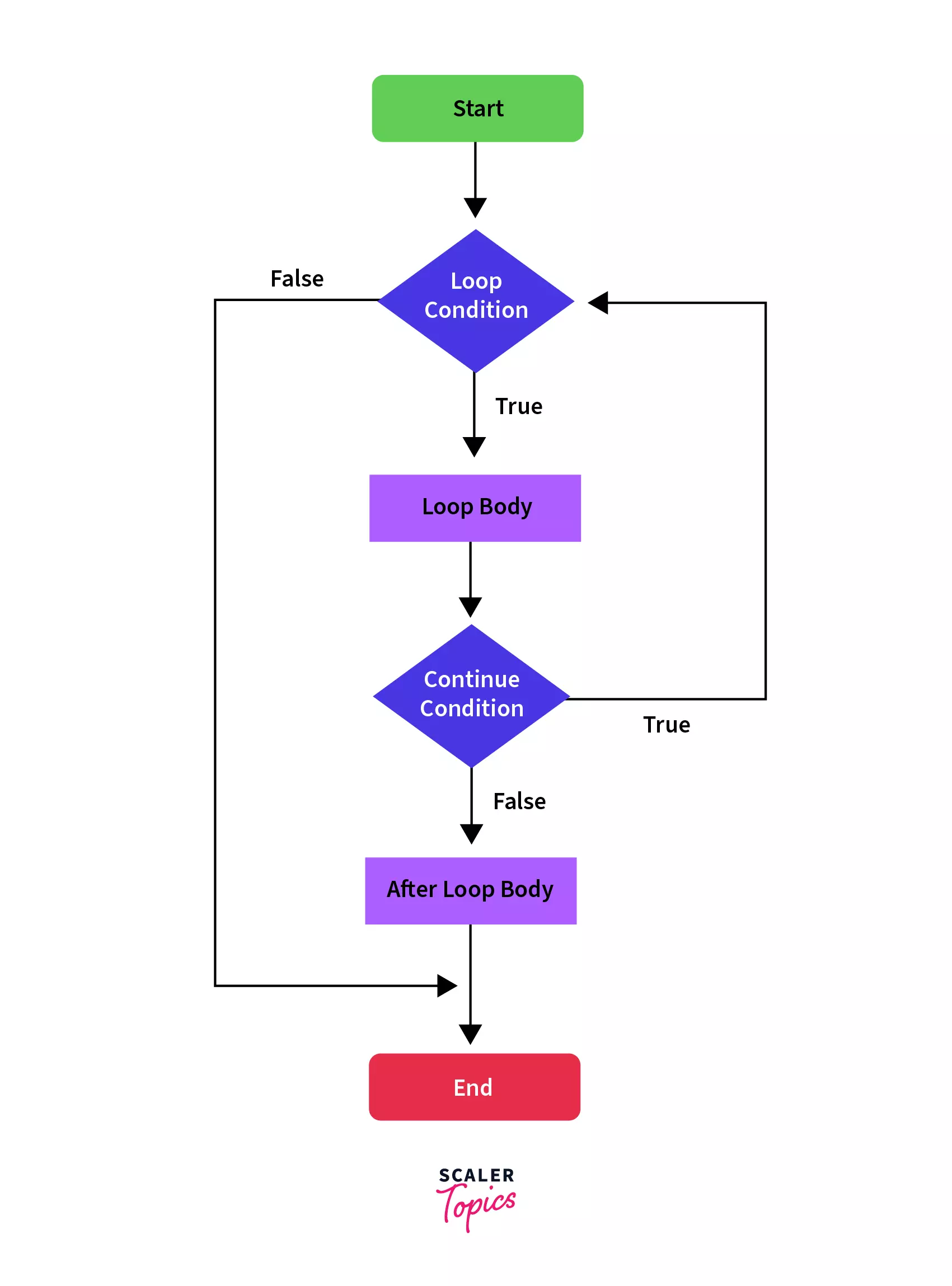In the above flow diagram of a continue statement, whenever the continue statement has encountered the rest of the loop body is skipped and the next iteration is executed if the loop condition is true.

The syntax and execution flow of the continue statement is as follows:

Syntax:

``````for(condition) {
// body of the loop
continue;
//the statements after this won't be executed
}
``````
``````while(condition) {
// body of the loop
continue;
// the statements after this won't be executed
}
``````

Example:

Let’s try to print the odd number between 1 to 10 as we did in the example of the for loop, but this time we’ll use the continue statement:

``````System.out.println("The odd numbers between 1 to 10 are: ");
for (int number = 1; number <= 10; number++) {
if (number % 2 == 0) continue;
System.out.println(number);
}
``````

Output:

``````The odd numbers between 1 to 10 are:
1
3
5
7
9
``````

### 3. Return Statement

The return statements are used when we need to return from a method explicitly. The return statement transfers the control back to the caller method of the current method. In the case of the main method, the execution is completed and the program is terminated. Return statements are often used for conditional termination of a method or to return something from the method to the caller method.

Let's see the execution flow of the return statement in a flow diagram: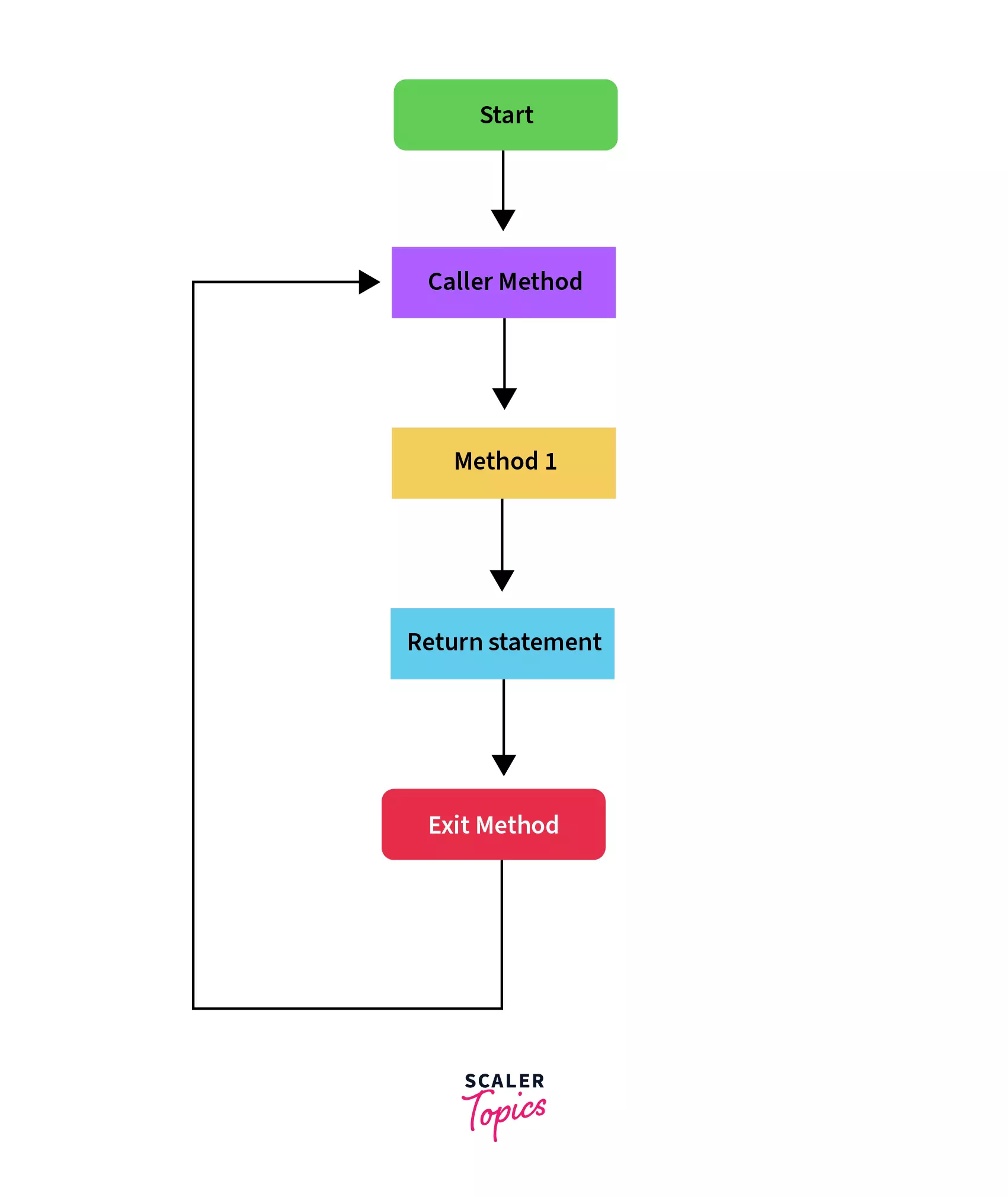As you can see in the above flow diagram, whenever a return statement is encountered anywhere in a method, the execution of the current method is stopped and the flow of the program returns to the caller method of the current method.

The syntax and execution flow of the return statement is as follows:

Syntax:

``````void method() {
// body of the method
return;
}
``````

Example:

Let’s say we are searching an element in a list, and as soon as we find it, our work is done and we should exit the function. Let’s see the implementation of this problem:

``````public class ReturnStatementDemo {

public static String search(int key) {
int[] numbers = { 1, 2, 3, 4, 5, 6, 7, 8, 9 };
for (int element : numbers) {
if (element == key) {
return "Success";
// Putting statements post this return statement
// Will throw compile-time error
}
}
return "Failure";
}

public static void main(String[] args) {
System.out.println(search(3));
System.out.println(search(10));
}
}
``````

Output:

``````Success
Failure
``````

As we can search that the value 3 was in the array and we get the output as “Success”, but since value 10 does not exist in the array, we get the “Failure” as the output.

## Conclusion

• Decision-making statements are used to control the flow based on certain conditions.
• There are various decision making statements in Java such if, if-else, if-else-if ladder and switch statements.
• Loop statements are used to run a block of code repeatedly a number of times.
• There are various loop statement constructs such as for, while, do-while and for-each statements.
• Branching statements are used to transfer the control of the program to a different block of code or method.
• There are various types of branching statements such as break, continue and return statments that transfer the control of execution to a specific point in the code.
• Control statements can make a Java program more effective and user-friendly when used efficiently. They help in implementing real world scenarios using programs.
Free Courses by top Scaler instructors